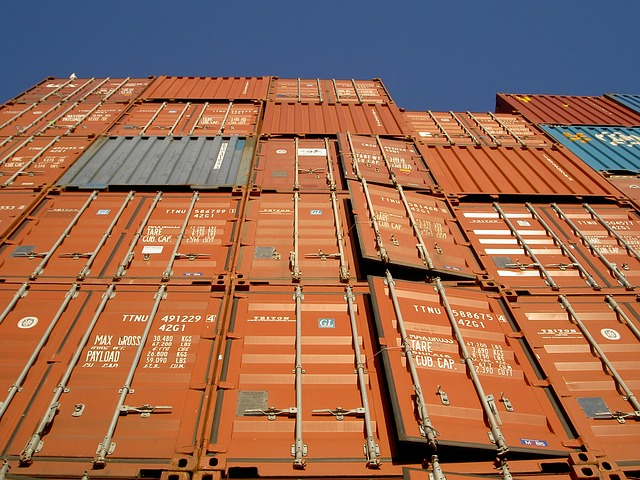# Comparing Surface Area & Volume

Student View (Opens in new window)# Lesson Overview

Students make two different rectangular prisms by folding two 8$\frac{1}{2}$ in. by 11 in. sheets of paper in different ways. Then students use reasoning to compare the total areas of the faces of the two prisms (i.e., their surface areas). Students also predict how the amounts of space inside the prisms (i.e., their volumes) compare. They will check their predictions in Lesson 6.

# Key Concepts

Students compare the total area of the faces (i.e., surface area) of one rectangular prism to the total area of the faces of another prism. Students make predictions about which prism has the greater amount of space inside (i.e., the greater volume). Students do not compute actual surface areas or volumes. This exploration helps pave the way for a more formal study of volume in Lesson 6 and a more formal study of surface area in Lesson 7.

# Goals and Learning Objectives

• Explore how the surface areas and volumes of two different prisms made from the same-sized sheet of paper compare.